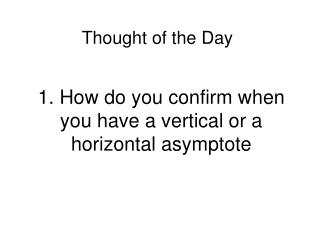DownloadDownload Presentation1. How do you confirm when you have a vertical or a horizontal asymptote

1. How do you confirm when you have a vertical or a horizontal asymptote

Download Presentation1. How do you confirm when you have a vertical or a horizontal asymptote

- - - - - - - - - - - - - - - - - - - - - - - - - - - E N D - - - - - - - - - - - - - - - - - - - - - - - - - - -
Presentation Transcript

1. Thought of the Day 1. How do you confirm when you have a vertical or a horizontal asymptote

2. Vertical – suspect them at x-values which cause the denominator of f(x) to be zero. Confirm that the limit as x a is infinite…. Horizontal – suspect rational functions Confirm that as x , y a

3. What is the definition of LIMIT? OK…this is like the basis of ALL of Calculus. It was finally “perfected” by Cauchy in 1821. Ready?

4. Given any if there is a corresponding such that implies then we say that lim (This is the bare bones important part that you need to memorize…check your text for the detailed version.)

5. How many different methods are there for evaluating limits? Can you name several?

6. Inspection • Observe graph • Create a table of values • Re-write algebraically • Use L’Hopitals Rule • (only if the form is indeterminate) • 6. Squeeze theorem (rarely used!!)

7. How many indeterminate forms can you name?

8. 1. 2. 3. 4. 5. 6. 7. Math Wars!!! Did you know all 7?

9. lim = ?

10. Zero! Zip…

11. What are the three main types of discontinuities?

12. Hole – at x=3 in the example • Step – usually the function’s description is split up : • Vertical asymptote – at x=1 in the example f(x)={ for x<0 for x>0

13. Under what conditions does the derivative NOT exist at x=a

14. If there is a discontinuity at x=a or if there is a sharp corner at x=a, then the derivative is undefined at x=a

15. What is the definition of continuity at a point?

16. What is a monotone function?

17. A function that is either always increasing or always decreasing. (i.e. the derivative is always positive or always negative.)